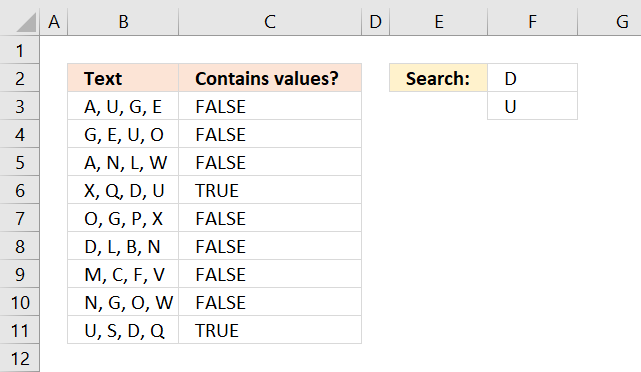Author: Oscar Cronquist Article last updated on October 31, 2018The array formula in cell C3 checks if text string in B3 contains all values in F2:F3.

=COUNT(SEARCH(\$F\$2:\$F\$3,B3))=2

To enter an array formula, type the formula in a cell then press and hold CTRL + SHIFT simultaneously, now press Enter once. Release all keys.

The formula bar now shows the formula with a beginning and ending curly bracket telling you that you entered the formula successfully. Don't enter the curly brackets yourself.

### Explaining formula in cell C3

The SEARCH function returns the character position of a substring in a text string, it returns an error if not found.

SEARCH(\$F\$2:\$F\$3,B3)

becomes

SEARCH({"D";"U"},"A, U, G, E")

and returns {#VALUE!;4}.

THE COUNT function counts the number of values that contain a number, it conveniently also ignores errors.

COUNT(SEARCH(\$F\$2:\$F\$3,B3))

becomes

COUNT({#VALUE!;4}) and returns 1. Only one value in the array is a number.

Lastly, the equal sign compares the output with number 2. COUNT(SEARCH(\$F\$2:\$F\$3,B3))=2

becomes

1=2 and returns FALSE in cell C3.

### Modify formula to check if at least one value is found

If you want the formula to return TRUE if at least one value is found change the formula to:

=COUNT(SEARCH(\$F\$2:\$F\$3,B3))=>1

The possibilities are endless here if you want the formula to return TRUE if at least 2 out of 3 values are found, change the formula to:

=COUNT(SEARCH(\$F\$2:\$F\$4,B3))=>2

### Regular formula

This formula is slightly larger but has an advantage of being a regular formula.

=SUMPRODUCT(COUNTIF(B3,"*"&\$F\$2:\$F\$3&"*")*1)=2